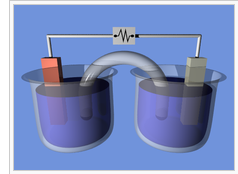## Electrochemical Cell Notation

#### Learning Objective

• Produce the appropriate electrochemical cell notation for a given electrochemical reaction

#### Key Points

• The cell anode and cathode (half-cells) are separated by two bars or slashes, which represent a salt bridge.
• The anode is placed on the left and the cathode is placed on the right.
• Individual solid, liquid, or aqueous phases within each half-cell are written separated by a single bar.
• Concentrations of dissolved species can be written in the parentheses after the phase notation (s, l, g, or aq).

#### Terms

• electrodeThe terminal through which electric current passes between metallic and nonmetallic parts of an electric circuit. In electrolysis, the electrodes are placed in the solution separately.
• half-cellEither of the two parts of an electrochemical cell containing an electrode and an electrolyte.

## Cell Notation

Recall that standard cell potentials can be calculated from potentials E0cell for both oxidation and reduction reactions. A positive cell potential indicates that the reaction proceeds spontaneously in the direction in which the reaction is written. Conversely, a reaction with a negative cell potential proceeds spontaneously in the reverse direction.

[latex]E^o _{cell} = E^o _{reduction} + E^o _{oxidation}[/latex]

Cell notations are a shorthand description of voltaic or galvanic (spontaneous) cells. The reaction conditions (pressure, temperature, concentration, etc.), the anode, the cathode, and the electrode components are all described in this unique shorthand.

Recall that oxidation takes place at the anode and reduction takes place at the cathode. When the anode and cathode are connected by a wire, electrons flow from anode to cathode.A typical galvanic cellA typical arrangement of half-cells linked to form a galvanic cell.

Using the arrangement of components, let’s put a cell together.

One beaker contains 0.15 M Cd(NO3)2 and a Cd metal electrode. The other beaker contains 0.20 M AgNO3 and a Ag metal electrode. The net ionic equation for the reaction is written:

[latex]2Ag^+ (aq) + Cd (s) \rightleftharpoons Cd^{2+} (aq) + 2Ag (s)[/latex]

In the reaction, the silver ion is reduced by gaining an electron, and solid Ag is the cathode. The cadmium is oxidized by losing electrons, and solid Cd is the anode.

The anode reaction is:

[latex]Cd (s) \rightleftharpoons Cd^{2+} (aq) + 2e^-[/latex]

The cathode reaction is:

[latex]2Ag^+ (aq) + 2e^- \rightleftharpoons 2Ag (s)[/latex]

## Cell Notation Rules

1. The anode half-cell is described first; the cathode half-cell follows. Within a given half-cell, the reactants are specified first and the products last. The description of the oxidation reaction is first, and the reduction reaction is last; when you read it, your eyes move in the direction of electron flow. Spectator ions are not included.

2. A single vertical line ( | ) is drawn between two chemical species that are in different phases but in physical contact with each other (e.g., solid electrode | liquid with electrolyte). A double vertical line ( || ) represents a salt bridge or porous membrane separating the individual half-cells.

3. The phase of each chemical (s, l, g, aq) is shown in parentheses. If the electrolytes in the cells are not at standard conditions, concentrations and/or pressure, they are included in parentheses with the phase notation. If no concentration or pressure is noted, the electrolytes in the cells are assumed to be at standard conditions (1.00 M or 1.00 atm and 298 K).

Using these rules, the notation for the cell we put together is:

Cd (s) | Cd2+ (aq, 0.15 M) || Ag+ (aq, 0.20 M) | Ag (s)